# python图像常规操作

PIL简介：

PIL(Python Imaging Library Python,图像处理类库)提供了通用的图像处理功能,以及大量有用的基本图像操作,比如图像缩放、裁剪、旋转、颜色转换等。

PIL读取与存储图像：

```# -*- coding: utf-8 -*-
from PIL import Image
import os
#打开图像得到一个PIL图像对象
img = Image.open("./source/test.jpg")
#将其转为一张灰度图
img = img.convert('L')
#存储该张图片
try:
img.save("test.png")
except IOError:
print "cannot convert"```

Test.jpgTest.png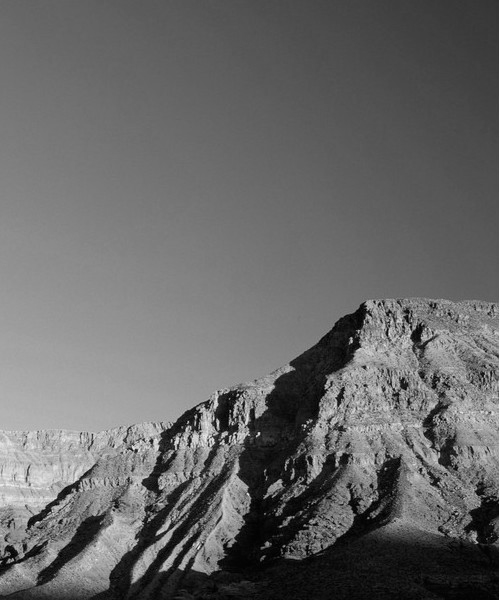PIL生成缩略图：

```# -*- coding: utf-8 -*-
from PIL import Image
import os
#打开图像得到一个PIL图像对象
img = Image.open("./source/test.jpg")
#创建最长边为128的缩略图
img.thumbnail((128,128))
#存储该张图片
try:
img.save("test.png")
except IOError:
print "cannot convert"```

Test.pngPIL调整尺寸与旋转：

```# -*- coding: utf-8 -*-
from PIL import Image
import os
#打开图像得到一个PIL图像对象
img = Image.open("./source/test.jpg")
#修改图片大小，参数为一元组
img = img.resize((100,200))
#使图片逆时针选择45度
img = img.rotate(45)
#存储该张图片
try:
img.save("test.png")
except IOError:
print "cannot convert"```

Test.pngPIL复制粘贴图像区域：

```# -*- coding: utf-8 -*-
# -*- coding: utf-8 -*-
from PIL import Image
import os
#打开图像得到一个PIL图像对象
img = Image.open("./source/test.jpg")
#从img中裁剪指定区域
region = img.crop((300,300,500,500))
#使裁剪部分逆时针选择145度
region = region.rotate(145)
#将该区域粘贴至指定区域
img.paste(region,(100,100,300,300));
#存储该张图片
try:
img.save("test.png")
except IOError:
print "cannot convert"```

Test.png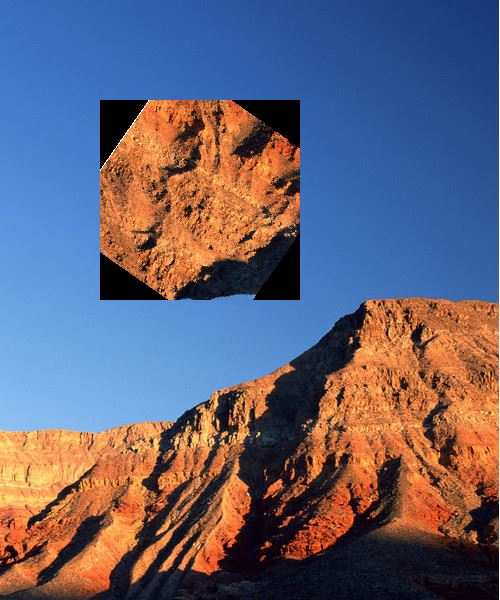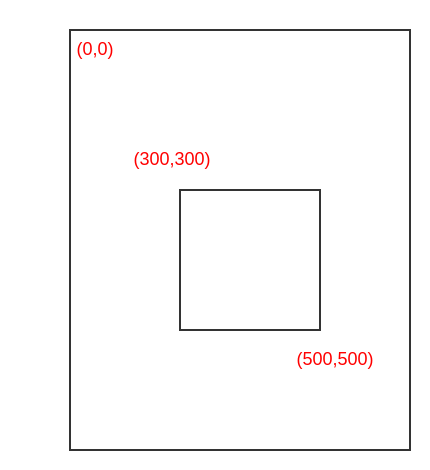Matplotlib简介：

```# -*- coding: utf-8 -*-
from PIL import Image
from pylab import *
#打开图像得到一个PIL图像对象
img = Image.open("./source/test.jpg")
# 读取图像到数组中
im = array(img)
# 绘制图像
imshow(im)
# 一些点
x = [100,100,400,400]
y = [200,500,200,500]
# 使用红色星状标记绘制点
plot(x,y,'r*')
# 绘制连接前两个点的线
plot(x[:2],y[:2])
# 添加标题,显示绘制的图像
title('Plotting: "Test.jpg"')
show()```

show() 命令首先打开图形用户界面(GUI),然后新建一个图像窗口。该图形用户界面会循环阻断脚本,然后暂停,直到最后一个图像窗口关闭。在每个脚本里,你只能调用一次 show() 命令,而且通常是在脚本的结尾调用。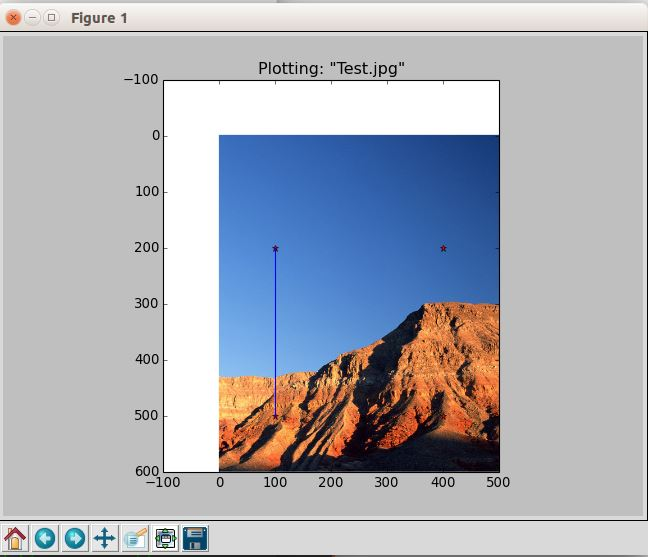```plot(x,y)      #默认为蓝色实线
plot(x,y,'r*')   #红色星状标记
plot(x,y,'go-')   #带有圆圈标记的绿线
plot(x,y,'ks:')   #带有正方形标记的黑色虚线```

‘b' 蓝色
‘g' 绿色
‘r' 红色
‘c' 青色
‘m' 品红
‘y' 黄色
‘k' 黑色
‘w' 白色

‘-‘ 实线
‘?' 虚线
‘:' 点线

‘.'
‘o' 圆圈
's' 正方形
‘*' 星形
‘+' 加号
‘x' 叉号

```# -*- coding: utf-8 -*-
from PIL import Image
from pylab import *
# 读取图像到数组中，并灰度化
im = array(Image.open('./source/test.jpg').convert('L'))
#显示时抛弃颜色信息
gray()
# 显示轮廓图像
contour(im, origin='image')
# 在原点的左上角显示
axis('equal')
#关闭坐标轴
axis('off')
show()```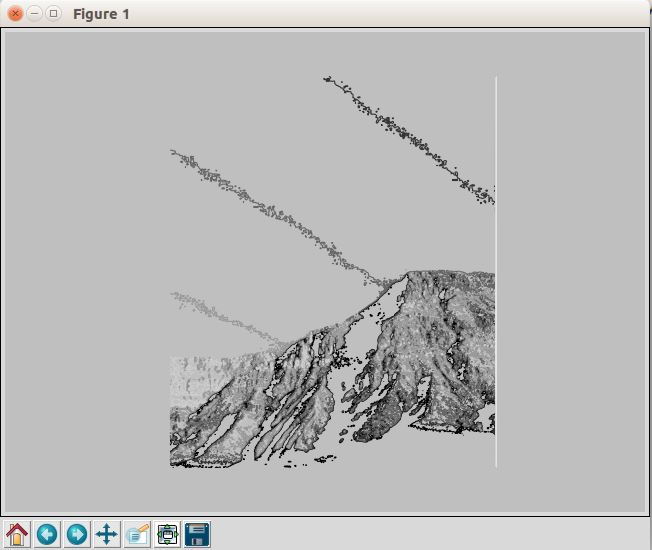hist() 函数的第二个参数指定小区间的数目。需要注意的是,因为 hist() 只接受一维数组作为输入,所以我们在绘制图像直方图之前,必须先对图像进行压平处理。flatten() 方法将任意数组按照行优先准则转换成一维数组。

```# -*- coding: utf-8 -*-
from PIL import Image
from pylab import *
# 读取图像到数组中，并灰度化
im = array(Image.open('./source/test.jpg').convert('L'))
# 直方图图像
hist(im.flatten(),128)
# 显示
show()```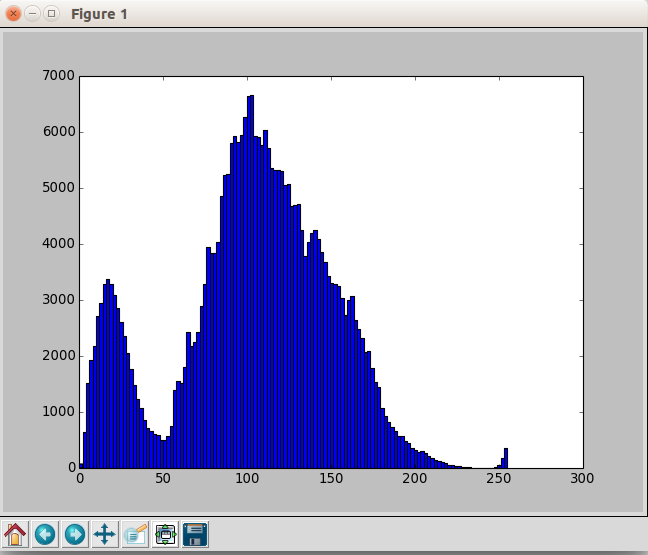PyLab 库中的 ginput() 函数可以实现交互式标注，用来标记一些点或者是一些训练数据。

```# -*- coding: utf-8 -*-
from PIL import Image
from pylab import *
# 读取图像到数组中
im = array(Image.open('./source/test.jpg'))
# 显示图像
imshow(im)
#获取点击并将点击坐标保存在[x,y]列表中
x = ginput(3)
#输出保存的数据
print 'you clicked:',x
show()```

`you clicked: [(295.22704081632651, 210.72448979591837), (405.43112244897952, 66.846938775510239), (439.1045918367347, 180.11224489795921)]`

python使用邻接矩阵构造图代码示例

python好玩的项目—色情图片识别代码分享

Python生成数字图片代码分享